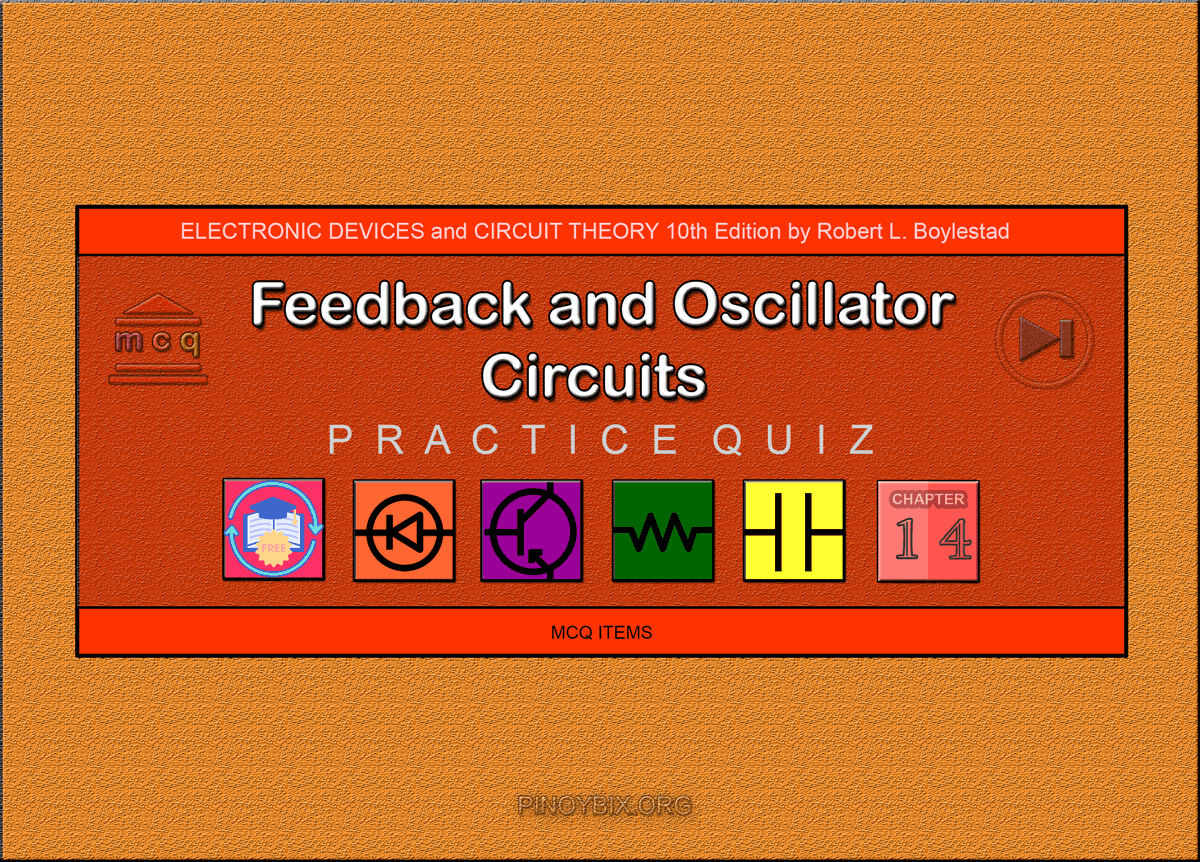(Last Updated On: February 4, 2020)Below are the answers key for the Multiple Choice Questions (Quiz) in Feedback and Oscillator Circuits from the book Electronic Devices and Circuit Theory 10th Edition by Robert L. Boylestad.

1. D. All of the above

2. D. All of the above

3. D. All of the above

4. A. 1 + βA

5. B. 1 + βA

6. A. True

7. B. –3.85

8. C. 390 kΩ

9. D. 769.23 Ω

10. B. 0.3%

11. A. –4.36

12. C. 4.75

13. C. –4.17

14. A. –20.0

15. B. –8.57

16. C. Both A and the phase shift between input and output signals

17. B. 180°

18. D. 0°

19. A. True

20. A. True

21. C. Both βA > 1 and the phase shift around the feedback network must be 180º.

22. B. False

23. C. 1

24. B. 8.48 kΩ

25. B. Greater than –29

26. A. phase-shift

27. B. 29

28. C. R1, R2, C1, and C2

29. A. 1560.34 Hz

30. D. 3558.8 Hz

31. C. 159 pF

32. B. Wien bridge

33. D. All of the above

34. C. Colpitts

35. A. 20,000

#### FILL-IN-THE-BLANKS

1. A. decreased voltage gain

2. C. oscillation in the circuit

3. B. increase, decrease

4. A. decrease, increase

5. D. 1 + βA

6. C. more

7. B. higher, smaller

8. A. Zero

9. C. increases

10. B. less than 1

11. D. 1

12. A. greater than 1

13. C. greater than 29

14. D. RC combinations

15. D. 2

16. D. both inductance and a capacitance

17. B. capacitors, inductor

18. A. inductors, capacitor

19. C. greater

20. D. high

21. A. very low

22. D. very high

23. A. very large

24. D. All of the above

25. C. 0.4, 0.6

### Complete List of Chapter Quiz in Electronic Devices and Circuit Theory by Boylestad

P inoyBIX educates thousands of reviewers and students a day in preparation for their board examinations. Also provides professionals with materials for their lectures and practice exams. Help me go forward with the same spirit.

“Will you subscribe today via YOUTUBE?”

Subscribe

© 2014 PinoyBIX Engineering. © 2019 All Rights Reserved | How to Donate? |